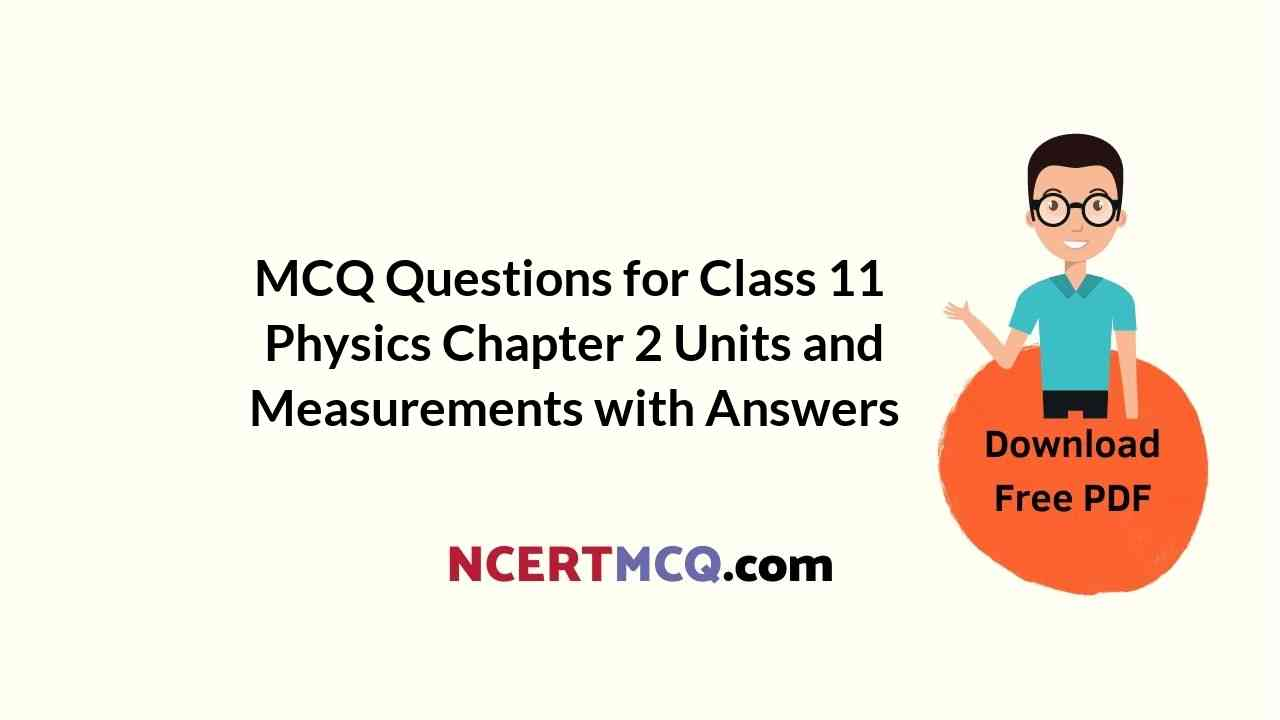Check the below NCERT MCQ Questions for Class 11 Physics Chapter 2 Units and Measurements with Answers Pdf free download. MCQ Questions for Class 11 Physics with Answers were prepared based on the latest exam pattern. We have provided Units and Measurements Class 11 Physics MCQs Questions with Answers to help students understand the concept very well.

## Units and Measurements Class 11 MCQs Questions with Answers

Units And Measurements Class 11 MCQ Question 1.
Physical quantities are
(b) quantities such as length, mass, time, electric current, thermodynamic temperature, amount of substance, and luminous intensity
(c) quantities such as pounds, dollars and rupees
(d) quantities such as kilos, pounds and gallons

Answer: (b) quantities such as length, mass, time, electric current, thermodynamic temperature, amount of substance, and luminous intensity

Class 11 Physics Chapter 2 MCQ Question 2.
Which of the following pairs has the same dimensions?
(a) specific heat and latent heat
(b) lmpulse and momentum
(c) surface tension and force
(d) moment of lnertia and torque

Unit And Measurement Class 11 MCQ Question 3.
The dimensions of kinetic energy is
(a) [M 2L2T]
(b) [ML 2T]
(c) [ML 2T-2]
(d) [ML 2T-1]

MCQ Questions For Class 11 Physics Chapter 2 Question 4.
A force F is given by F = at + bt², where t is time. What are the dimensions of a and b?
(a) MLT-1 and MLT0
(b) MLT-3 and ML2T4
(c) MLT-4 and MLT1
(d) MLT-3 and MLT-4

Class 11 Physics Chapter 2 MCQ With Answers Question 5.
The atmospheric pressure is 106 dyne/cm². What is its value in SI unit?
(a) 105 newton/m²
(b) 106 newton/m²
(c) 104 newton/m²
(d) 103 newton/m²

Class 11 Physics Ch 2 MCQ Question 6.
In a system of units if force (F), acceleration (A) and time (T) are taken as fundamentals units then the dimensional formula of energy is
(a) FA2T
(b) FAT2
(c) FA2T
(d) FAT

Ch 2 Physics Class 11 MCQ Question 7.
If force (F), work (W) and velocity (v) are taken as fundamental quantities. What is the dimensional formula of time (T)?
(a) [WFv]
(b) [WFv-1]
(c) [W-1F -1v]
(d) [WF-1v -1]

Physics Class 11 Chapter 2 MCQ Question 8.
The dimensions of kinetic energy is same as that of
(a) force
(b) pressure
(c) work
(d) momentum

MCQs Of Physics Class 11 Chapter 2 Question 9.
Which of the following groups have different dimensions?
(a) Potential difference, EMF, voltage
(b) Pressure, stress, Youngs modulus
(c) Heat, energy, work done
(d) Dipole moment, electric flux, electric field

Answer: (d) Dipole moment, electric flux, electric field

MCQ Class 11 Physics Chapter 2 Question 10.
ML-1T -2 is the dimensional formula of
(a) magnetic induction
(b) self-inductance
(c) electric potential
(d) electric field

Units And Measurements MCQ Question 11.
What is the dimensional formula of magnetic field?
(a) MT-2A-1
(b) MT-1A-2
(c) M-1L-2TA-1
(d) M-1LTA-2

MCQ On Units And Measurements Question 12.
Electron volt is a unit of
(a) charge
(b) potential difference
(c) energy
(d) magnetic force

MCQ Questions On Units And Measurements Class 11 Question 13.
The volume of a cube in m³ is equal to the surface area of the cube in m². The volume of the cube is
(a) 64 m³
(b) 216 m³
(c) 512 m³
(d) 196 m³

MCQ On Units And Measurements Class 11 Pdf With Answers Question 14.
In SI system the fundamental units are
(a) meter, kilogram, second, ampere, Kelvin, mole and candela
(b) meter, kilogram, second, coulomb, Kelvin, mole and candela
(c) meter, Newton, second, ampere, Kelvin, mole and candela
(d) meter, kilogram, second, ampere, Kelvin, mole and lux

Answer: (b) meter, kilogram, second, coulomb, Kelvin, mole and candela

Chapter 2 Physics Class 11 MCQ Question 15.
Which one of the following represents the correct dimensions of the coefficient of viscosity?
(a) [ML-1T-2]
(b) [MLT-1]
(c) [ML-1T-1]
(d) [ML-2T-2]

MCQ Of Units And Measurements Class 11 Question 16.
A particle starting from the origin (0, 0) moves in a straight line in the (x, y) plane. Its coordinates at a later time are the path of the particle makes with the x-axis an angle of
(a) 300
(b) 450
(c) 600
(d) 0

MCQ Questions For Class 11 Physics Chapter 1 Units And Measurements Question 17.
Resolution is
(a) a measure of the bias in the instrument
(b) None of these
(c) the smallest amount of input signal change that the instrument can detect reliably
(d) a measure of the systematic errors

Answer: (d) a measure of the systematic errors

Class 11 Units And Measurements MCQ Question 18.
Absolute error of the measurement is
(a) the difference between the individual measurement and the true value of the quantity cubed.
(b) the difference between the individual measurement and the true value of the quantity squared.
(c) the difference between two individual measurements and their mean
(d) the difference between the individual measurement and the true value of the quantity

Answer: (a) the difference between the individual measurement and the true value of the quantity cubed.

MCQ Physics Class 11 Chapter 2 Question 19.
Which of the following units denotes the dimensions [ML2/Q2], where Q represents the electric charge?
(a) Wb/m²
(b) Henry(H)
(c) H/m²
(d) Weber(Wb)

MCQ Of Chapter 2 Physics Class 11 Question 20.
Light year is a unit of
(a) time
(b) distance
(c) sunlight intensity
(d) mass